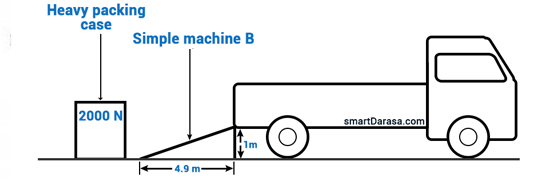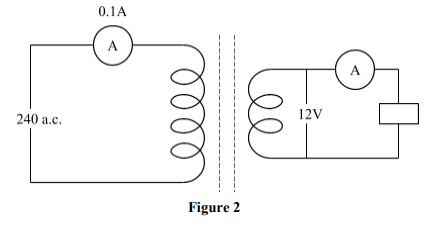## NECTA QNS

Type keyword | Title of the question to get it easly:

• PHYSICS | NECTA 2019 | Question 05

(a) Figure 1 shows a simple machine B which has to be used to pull the packing case of 2000 N into the car by an effort of 500 N. Calculate the efficiency of machine B.(b) With the aid of a clearly labelled diagram, describe an experiment to investigate the relationship between the force acting on a body and the acceleration produced.

• PHYSICS | NECTA 2019 | Question 08

(a) Why the inner core of the earth is solid while the outer core is liquid? Briefly explain.
(b) The frequency obtained from a plucked string when the tension is 2 N is 400 Hz. Calculate the frequency when the tension is increased by 6 N.

• PHYSICS | NECTA 2018 | Question 10

(a) State the following rules:
(i) Cork screw rule.
(ii) Dynamo rule.

(b)(i) Give one structural difference between A.C. and D.C. generators.
(ii) Mention one application of induction coil.

(c) Figure 2 shows a transformer used to step down power. Assuming that there are no power losses, what will be the ammeter reading on the output part.• PHYSICS | NECTA 2018 | Question 07

(a) What is meant by radioactive decay?

(b) A certain sample with half-life of 8 days contains 16 g of iodine 131.
(i)Write an expression to show the decay process of the sample.
(ii) Use an expression in (b) (i) to sketch the graph then estimate the mass of sample which will remain undecayed after 20 days.

(c) Describe the use of Geiger-Muller (G-M) tube in detecting nuclear radiations.

• PHYSICS | NECTA 2017 | Question 11

(a)(i) How does the increase of length and cross-section area of a conductor affect its resistance?

(b)(i) State the function of a circuit breaker in a wiring system.
(ii) Determine the ratio of resistance of wire A to that of wire B which are made up of the same material such that wire A has half the length and twice the diameter of wire B.

(c) An electric kettle contains 720 W heating units:
(i) What current does it take from 240 V mains?
(ii) How long will the kettle take to raise the temperature of 2 kg of water at 30°C to its boiling point?

• PHYSICS | NECTA 2017 | Question 06
(a)(i) What peculiar property does the effort has in all classes of levers?
(ii) A meter rule of weight 1.0N is supported horizontally on two knife edges each placed 10.0cm from its ends. If the weight of 1.5N is placed at its mid-point, calculate the reaction at the supports.

(b)(i) State the law of floatation.
(ii) Find the fraction of the cork that partially immersed when a piece of cork of density 0.25 g/cm3 and a mass of 20 g floats in water.

• PHYSICS | NECTA 2016 | Question 07

(a) What is meant by the terms:
(i) Bimetallic strip
(ii) Linear expansivity of the solid

(b)(i) List two factors that affects the stability of a body
(ii) Briefly explain why the handle of the door is near its outside edge?

(c) Briefly explain on the following observations:
(i) In cold weather the metal blade of a knife feels cooler than the wooden handle.
(ii) A cool breeze blows from the sea on a hot summer day.

• PHYSICS | NECTA 2016 | Question 04

(a)(i) Define the turning effect of force and give its SI Unit.
(ii) How can the moment of force be increased considerably in practical life? Give two examples.

(b)(i) List two factors that affects the stability of a body
(ii) Briefly explain why the handle of the door is near its outside edge?

(c) (i) What is meant by a balanced beam?
(ii)A uniform rod AB of mass 6.0g is balanced horizontally about a knife edge at a distance of 3cm form end A where a mass of 8.0g is hanging. Find the length of the rod.

• PHYSICS | NECTA 2015 | Question 10

(a)(i) List four main parts of a ripple tank.
(ii) What role does a stroboscope play in a ripple tank experiment?

(b)(i) Explain why there are four strings of different thickness in a violin?
(ii) What does a violinist do to change the note emitted by a particular string?

(c)(i) Briefly explain how a resonance tube works
(ii) Calculate the frequency of vibration in a resonance tube of shortest length of 0.22m when the next resonance length is 0.47m.

• PHYSICS | NECTA 2015 | Question 06

(a)(i) State two ways in which the image formed in plane mirror differ from that in a pin hole camera.
(ii) What is the effect of moving the pinhole camera closer to the object?

(b)(i) List three rules used to locate images in curved mirrors
(ii) Give two similarities and two differences that exist between the human eye and lens camera

(c) (i) A mirage is often seen by a motorist as a pool of water on the road some distance ahead;
(i)Draw a sketch diagram to show the formation of such a mirage.
(ii)Briefly explain how mirage is formed.